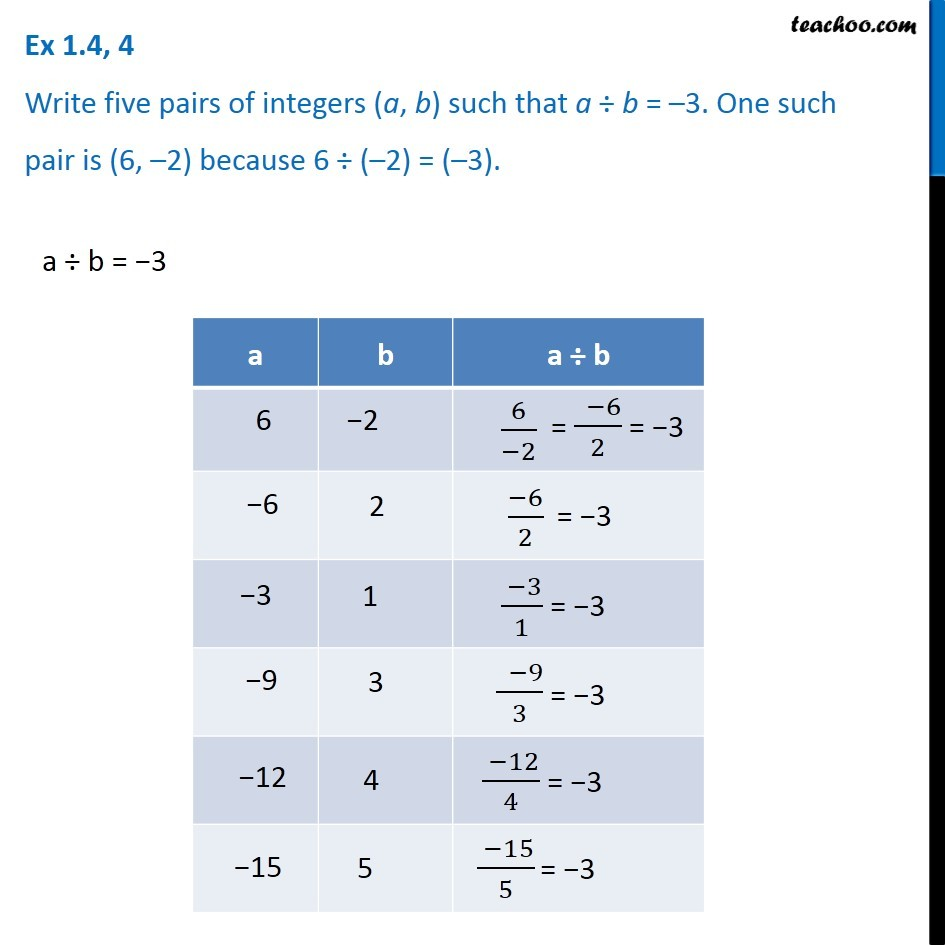1. Chapter 1 Class 7 Integers
2. Concept wise
3. Division of Integers

Transcript

Ex 1.4, 4 - Chapter 1 Class 7 Integers - NCERT Book Write five pairs of integers (a, b) such that a ÷ b = –3. One such pair is (6, –2) because 6 ÷ (–2) = (–3). a ÷ b = −3 So, pairs can be (6, -2) because because 6 ÷ (-2) = -6/2 = (-3) (-6, 2) because because (-6) ÷ 2 = (-3) (-3, 1) because because (-3) ÷ 1 = (-3) (-9, 3) because because (-9) ÷ 3 = (-3) (-12, 4) because because (-12) ÷ 4 = (-3) (-15, 5) because because (-15) ÷ 5 = (-3) Thus, pairs are (6,-2), (-6,2), (-3,1), (-9,3), (-12,-4), (-15,-5)